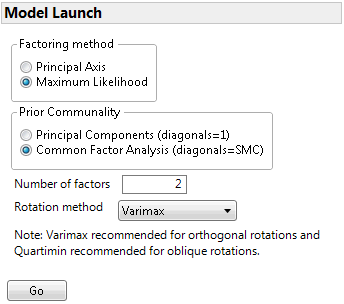Publication date: 05/24/2021

## Model Launch

Configure the Factor Analysis from the Model Launch control panel. Click Go to obtain the results of the factor analysis.

Figure 9.6 Model LaunchFactoring method

Defines the method for extracting factors:

Principal Axis

Performs eigenvalue decomposition on a reduced correlation or covariance matrix, where the diagonal of the matrix is replaced by an estimate of the communality of the variables. This method is a computationally efficient method, but it does not allow for hypothesis testing.

Maximum Likelihood

Enables you to test hypotheses about the number of common factors and to obtain model fit statistics.

Note: The Maximum Likelihood method requires a positive definite correlation matrix. If your correlation matrix is not positive definite, select the Principal Axis method.

Prior Communality

Defines the method for estimating the proportion of variance contributed by common factors for each variable.

Principal Components (diagonals = 1)

Sets all communalities equal to 1, indicating that 100% of each variable’s variance is explained by all of the factors.

Tip: Using this option with Factoring Method set to Principal Axis results in principal component analysis.

Common Factor Analysis (diagonals = SMC)

Sets the communalities equal to squared multiple correlation (SMC) coefficients. For a given variable, the SMC is the RSquare for a regression of that variable on all other variables.

Number of factors

Specifies the number of factors to extract from the analysis. The default is the number of eigenvalues that are greater than or equal to 1.0. You can set the number of factors to at least one and no more than the number of variables.

Rotation method

Defines the method for rotation. The default is Varimax. See Rotation Methods for a description of the available rotation methods.